# Problems 4-9 refer to this same capacitor: The plates of a parallel plate capacitor each have...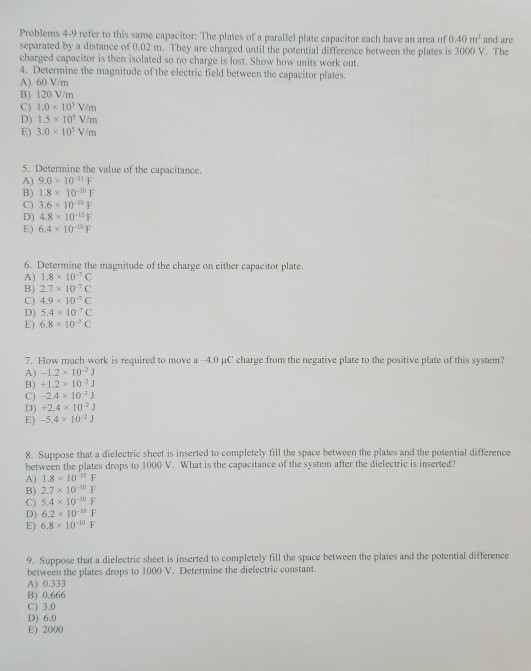Problems 4-9 refer to this same capacitor: The plates of a parallel plate capacitor each have an area of 0.40 m and are separated by a distance of 0.02 m. They are charged until the potential difference between the plates is 3000 V. The charged capacitor is then isolated so no charge is lost. Show how units work out. 4. Determine the magnitude of the electric field between the capacitor plates. A) 60 V/m B) 120 V/m C) 1.0 x 10s V/m D) 1.5 x 10 V/m E) 3.0 x 10 V/m 5. Determine the value of the capacitance. A) 9.0× 10-11 F B) 1.8× 10-10F C) 3.6-10-10F D) 4.8 x 10-10F E) 6.4× 10-10F 6. Determine the magnitude of the charge on either capacitor plate. A) 1.8 x 10 C B) 2.7 107 C C) 4.9 10"C D) 5.4 10 c E) 6.8 × 10-3 C 7. How much work is required to move a -4.0 uC charge from the negative plate to the positive plate of this system? A) -1.2 x 102J B) +1.2 x 10 J C)-2.4× 10-リ D) +2.4 x 102 E)-5.4× 10-2 J 8. Suppose that a dielectric sheet is inserted to completely fill the space between the plates and the potential difference between the plates drops to 1000 V. What is the capacitance of the system after the dielectric is inserted? A) 1.8 x 10t0 F B) 2.7 x 1010 F C) 5.4 x 10-10 F D) 6.2 x 10-10 F E) 6.8 10-10 F 9. Suppose that a dielectric sheet is inserted to completely fill the space between the plates and the potential difference between the plates drops to 1000 V. Determine the dielectric constant A) 0.333 B) 0.666 C) 3.0 D) 6.0 E) 2000

4. E = V / d = (3000 V) / 0.02 m

E = 1.5 x 10^5 V/m

Ans(D)

5. C = e0 A / d = (8.854 x 10^-12)(0.40)/0.02

C = 1.8 x 10^-10 F

Ans(B)

6. Q = C V = (1.8 x 10^-10)(3000) = 5.4 x 10^-7 C

Ans(D)

7. W = q delta(V) = (-4 x 10^-6)(0 - 3000)

W = 1.2 x 10^-3 J

Ans(B)

8. Q = C V = constant

C = (1.8 x 10^-10)(3000)/1000

C = 5.4 x 10^-10 F

Ans(C)

9. k = Cf/ Ci = 5.4/1.8 = 3

Ans(C)

#### Earn Coin

Coins can be redeemed for fabulous gifts.

Similar Homework Help Questions
• ### A parallel plate capacitor is made of plates of area 0.05 m? each. The plates are...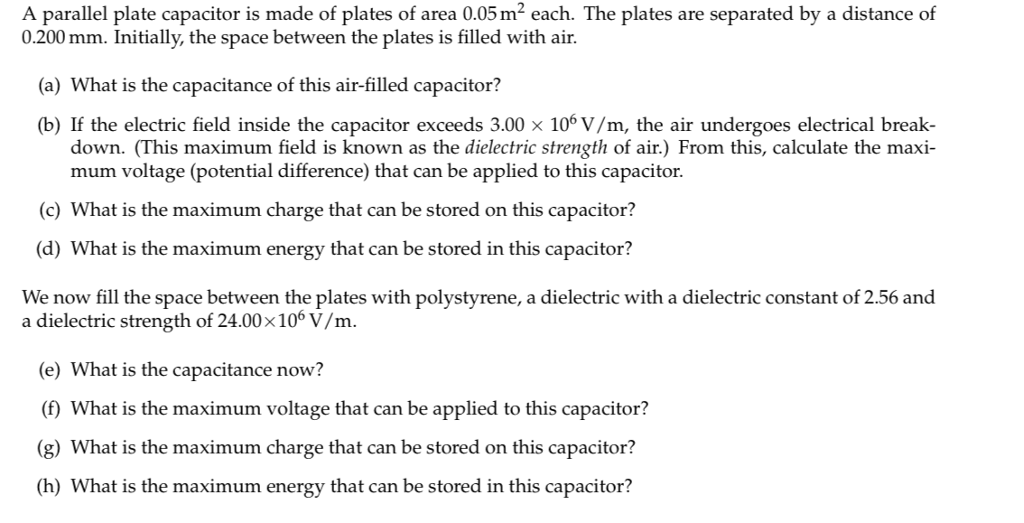A parallel plate capacitor is made of plates of area 0.05 m? each. The plates are separated by a distance of 0.200 mm. Initially, the space between the plates is filled with air. (a) What is the capacitance of this air-filled capacitor? (b) If the electric field inside the capacitor exceeds 3.00 x 106 V/m, the air undergoes electrical break- down. (This maximum field is known as the dielectric strength of air.) From this, calculate the maxi- mum voltage (potential...

• ### A parallel-plate capacitor has charge of magnitude 8.40 µC on each plate and capacitance 3.60 µF...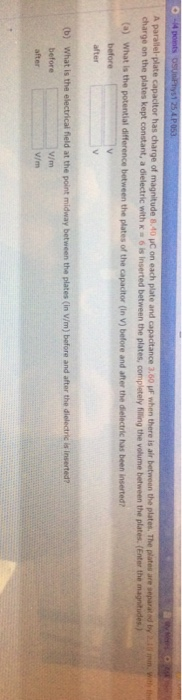A parallel-plate capacitor has charge of magnitude 8.40 µC on each plate and capacitance 3.60 µF when there is air between the plates. The plates are separated by 2.10 mm. With the charge on the plates kept constant, a dielectric with κ = 6 is inserted between the plates, completely filling the volume between the plates. (Enter the magnitudes.) (a) What is the potential difference between the plates of the capacitor (in V) before and after the dielectric has been...

• ### A parallel-plate capacitor has a plate area of A = 250 cm2 and a separation of...

A parallel-plate capacitor has a plate area of A = 250 cm2 and a separation of d = 2.00 mm. The capacitor is charged to a potential difference of V0 = 150 V by a battery. A dielectric sheet (κ = 3.50) of the same area but thickness ℓ = 1.00 mm is placed between the plates without disconnecting the battery. (See figure 24-18 on page 642). Determine the initial capacitance of the air-filled capacitor. Determine the charge on the...

• ### 24.26 Parallel-Plate Capacitor

A parallel-plate capacitor has capacitance C=12.5pF when the volume between the plates is filled with air. The plates are circular, with radius 3.00 cm. The capacitoris connected to a battery and a charge of magnitude 25.0 pC goes onto each plate. With the capacitor still connected to the battery, a slab of dielectric is insertedbetween the plates, completely filling the space between the plates. After the dielectric has been inserted, the charge on each plate has magnitude 45.0 pC.a.) what...

• ### Suppose the parallel plates in Fig. 24.15 each have an area of 2000 cm2 (2.00 x 101m2) and are 1....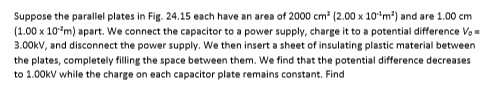Suppose the parallel plates in Fig. 24.15 each have an area of 2000 cm2 (2.00 x 101m2) and are 1.00 cm (1.00 x 104m) apart, we connect the capacitor to a power supply, charge it to a potential difference V。. 3.00kv, and disconnect the power supply. We then insert a sheet of insulating plastic material between the plates, completely filling the space between them. We find that the potential difference decreases to 1.00kV while the charge on each capacitor plate...

• ### A parallel-plate capacitor has capacitance Cq = 7.00 pF when there is air between the plates....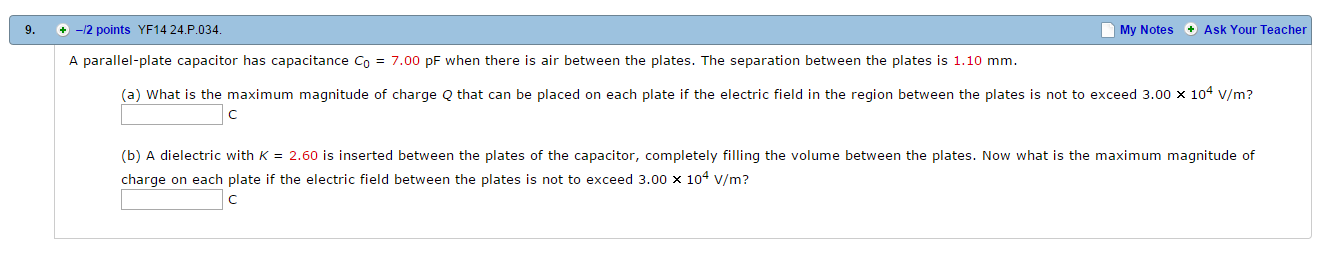A parallel-plate capacitor has capacitance Cq = 7.00 pF when there is air between the plates. The separation between the plates is 1.10 mm. What is the maximum magnitude of charge Q that can be placed on each plate if the electric field in the region between the plates is not to exceed 3.00 x 10^4 V/m? A dielectric with K = 2.60 is inserted between the plates of the capacitor, completely filling the volume between the plates. Now what...

• ### A parallel-plate capacitor has a 4 mm plate separation, 0.5 m^2 surface area per plate, and...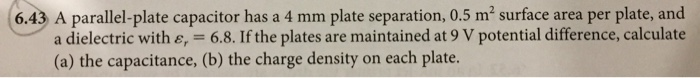A parallel-plate capacitor has a 4 mm plate separation, 0.5 m^2 surface area per plate, and a dielectric with E_r = 6.8. If the plates are maintained at 9 V potential difference, calculate the capacitance, the charge density on each plate.

• ### A parallel-plate capacitor has a plate area of A = 250 cm2 and a separation of...

A parallel-plate capacitor has a plate area of A = 250 cm2 and a separation of d = 2.00 mm. The capacitor is charged to a potential difference of V0 = 150 V by a battery. A dielectric sheet (κ = 3.50) of the same area but thickness ℓ = 1.00 mm is placed between the plates without disconnecting the battery. (See figure 24-18 on page 642). Determine the electric field in the dielectric. Determine the free charge on the...

• ### A parallel plate capacitor has an area A = 20.0 cm2 and a plate separation of d = 4.00 mm

A parallel plate capacitor has an area A = 20.0 cm2 and a plate separation of d = 4.00 mm.(a) Find the capacitance in air and the maximum voltage and charge the capacitor can hold.(b) A Teflon sheet is slid between the plates, filling the entire volume, Find the new capacitance and maximum charge.(c) Before the insertion of the Teflon, the plates are connected to a 24.0 V battery and then disconnected. What are the energies in the capacitor before...

• ### A parallel-plate capacitor (Homework #2) A parallel-plate capacitor is made by a pair of plates with...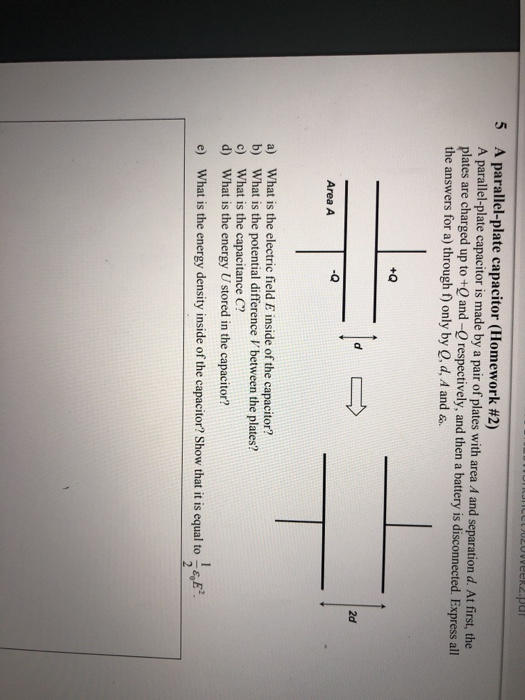A parallel-plate capacitor (Homework #2) A parallel-plate capacitor is made by a pair of plates with area A and separation d. At first, the plates are charged up to +Q and -O respectively, and then a battery is disconnected. Express all the answers for a) through f) only by Q, d, A and 5 +Q 2d -Q Area A a) What is the electric field E inside of the capacitor? b) What is the potential difference V between the plates?...

Free Homework App